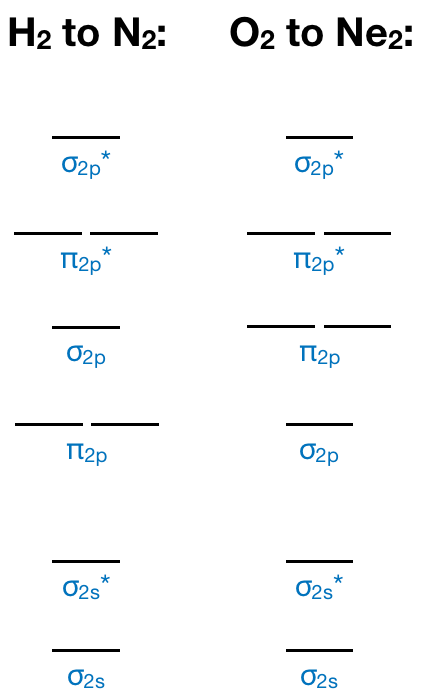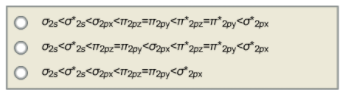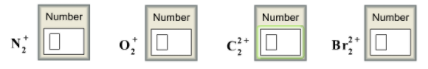# Problem: What is the valence molecular orbital configuration (according to MO theory) of homonuclear diatomic species where the atoms have an atomic number greater than seven? (Note that here we are distinguishing degenerate orbitals from each other where the two π2p's are shown as π2pz = π2py) How many electrons are there in the π antibonding orbitals of the following captions?

###### FREE Expert Solution

Recall that we have two types of MO diagrams:83% (337 ratings)###### Problem Details

What is the valence molecular orbital configuration (according to MO theory) of homonuclear diatomic species where the atoms have an atomic number greater than seven? (Note that here we are distinguishing degenerate orbitals from each other where the two π2p's are shown as π2pz = π2py)How many electrons are there in the π antibonding orbitals of the following captions?